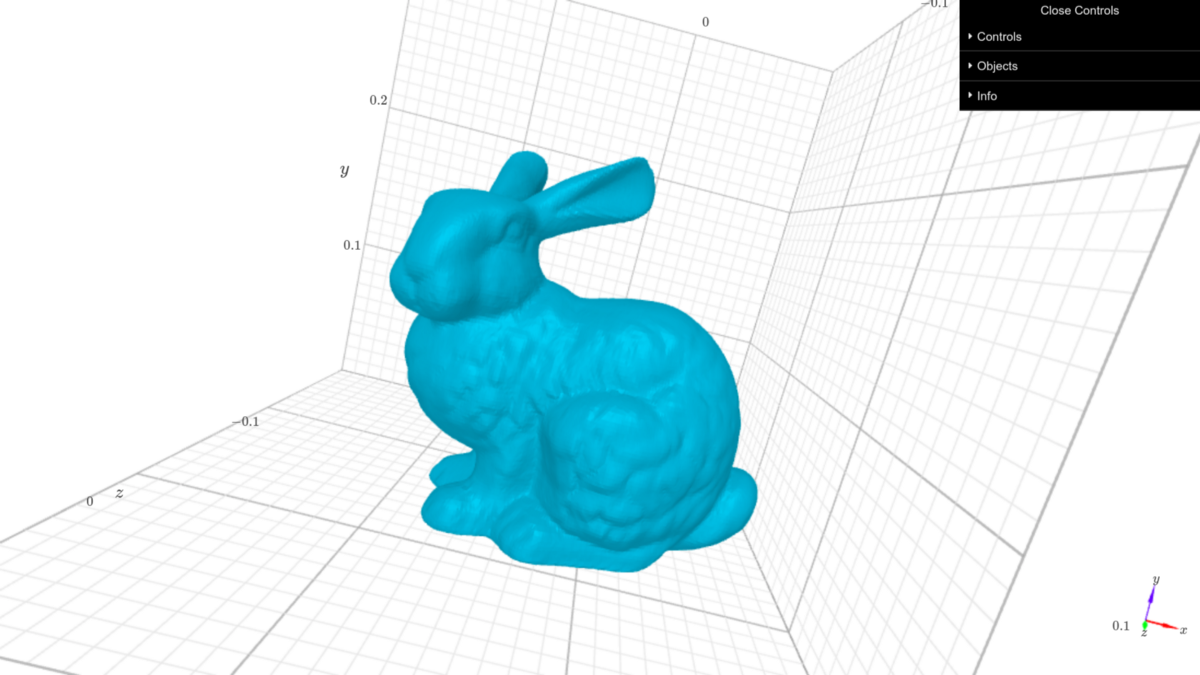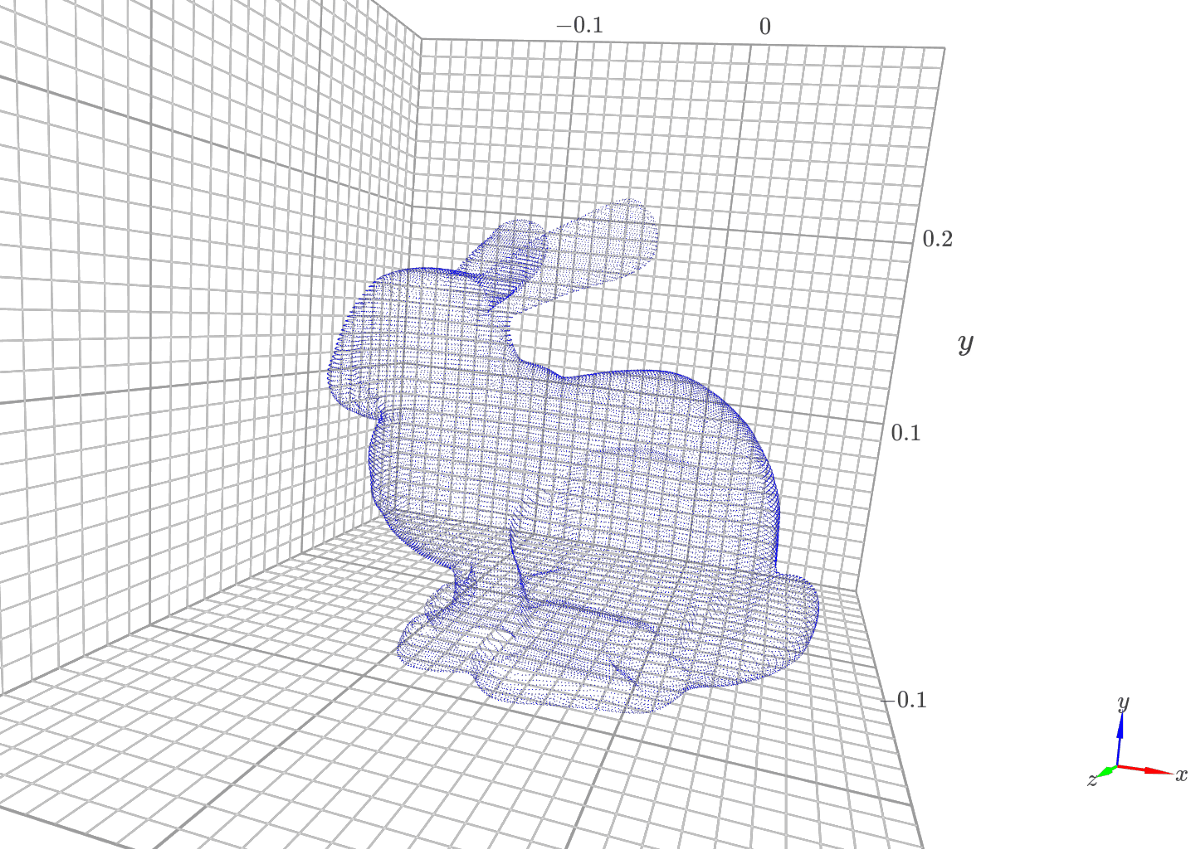# 如何使用Python Multiprocessing库处理3D数据？使用Python Multiprocessing库处理3D数据方法！

``````from io import BytesIO
import py7zlib

class MeshesArchive(object):
def __init__(self, archive_path):
fp = open(archive_path, 'rb')
self.archive = py7zlib.Archive7z(fp)
self.archive_path = archive_path
self.names_list = self.archive.getnames()
self.cur_id = 0

def __len__(self):
return len(self.names_list)

def get(self, name):
return bytes_io

def __getitem__(self, idx):
return self.get(self.names[idx])

def __iter__(self):
return self

def __next__(self):
if self.cur_id >= len(self.names_list):
raise StopIteration
name = self.names_list[self.cur_id]
self.cur_id += 1
return self.get(name)``````

``````class Archive7z(Base):
# ...
self.files = {}
# ...
for info in files.files:
# create an instance of ArchiveFile that knows location on disk
file = ArchiveFile(info, pos, src_pos, folder, self, maxsize=maxsize)
# ...
self.files.append(file)
# ...
self.files_map.update([(x.filename, x) for x in self.files])

# method that returns an ArchiveFile from files_map dictionary
def getmember(self, name):
if isinstance(name, (int, long)):
try:
return self.files[name]
except IndexError:
return None

return self.files_map.get(name, None)

class Archive7z(Base):
# ...
for level, coder in enumerate(self._folder.coders):
# ...
# get the decoder and decode the underlying data
data = getattr(self, decoder)(coder, data, level, num_coders)

return data``````

``````import trimesh
import k3d

with open("./data/meshes/stanford-bunny.obj") as f:

plot = k3d.plot()
mesh = k3d.mesh(bunny_mesh.vertices, bunny_mesh.faces)
plot += mesh
plot.display()``````k3d 显示的斯坦福兔子网格

``````import trimesh
import k3d

with open("./data/meshes/stanford-bunny.obj") as f:

plot = k3d.plot()
plot += cloud
plot.display()``````k3d绘制的

`map``imap`影响我们的区别在于，`map`在将其发送到工作进程之前将其转换为列表。如果存档太大而无法写入 RAM，则不应将其解压缩到 Python 列表中。换句话说，两者的执行速度是相似的。

``````[加载网格：pool.map w/o manager] 4 个进程池耗时：37.213207403818764 秒
[加载网格：pool.imap_unordered w/o manager] 4 个进程池耗时：37.219303369522095 秒``````

``````import numpy as np
from numpy.random import default_rng

def normalize_pc(points):
points = points - points.mean(axis=0)[None, :]
dists = np.linalg.norm(points, axis=1)
scaled_points = points / dists.max()
return scaled_points

STD = 1e-3
with open(bunny_path) as f:
# normalize point cloud
scaled_bunny = normalize_pc(bunny_mesh.vertices)
# add some noise to point cloud
rng = default_rng()
noise = rng.normal(0.0, STD, scaled_bunny.shape)
distorted_bunny = scaled_bunny + noise
return distorted_bunny``````

``````import itertools
import time
import numpy as np
from numpy.random import default_rng
import trimesh
import igl
from tqdm import tqdm
from multiprocessing import Pool
return mesh
def get_max_dist(base_mesh, point_cloud):
distance_sq, mesh_face_indexes, _ = igl.point_mesh_squared_distance(
point_cloud,
base_mesh.vertices,
base_mesh.faces
)
return distance_sq.max()
obj_file, point_cloud = args, args
mesh.vertices = normalize_pc(mesh.vertices)
max_dist = get_max_dist(mesh, point_cloud)
return max_dist
# do the meshes processing within a pool
elapsed_time = []
for _ in range(num_iterations):
archive = MeshesArchive(archive_path)
pool = Pool(num_proc)
start = time.time()
result = list(tqdm(pool.imap(
zip(archive, itertools.repeat(point_cloud)),
), total=len(archive)))
pool.close()
pool.join()
end = time.time()
elapsed_time.append(end - start)
print(f'[Process meshes: pool.imap] Pool of {num_proc} processes elapsed time: {np.array(elapsed_time).mean()} sec')
for name, dist in zip(archive.names_list, result):
print(f"{name} {dist}")
return result
if __name__ == "__main__":
bunny_path = "./data/meshes/stanford-bunny.obj"
archive_path = "./data/meshes.7z"
num_proc = 4
num_iterations = 3

• MeshesArchive并multiprocessing.Pool初始化
• tqdm 用于观察池进度，并手动完成整个池的分析
• 执行结果的输出

``````100%|########################################### #####################| 5/5 [00:00<00:00, 5.14it/s]
100%|########################### ####################################| 5/5 [00:00<00:00, 5.08it/s]
100%|########################### ####################################| 5/5 [00:00 <0时，5.18it /秒]
[方法网眼：pool.imap W / O管理器] 4个过程的池经过时间：1.0080536206563313秒